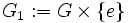Normal not implies characteristic in the collection of all groups satisfying a nontrivial finite direct product-closed group property

Statement

Suppose$\mathcal{V}$ is the subcollection of the collection of all groups that satisfies a finite direct product-closed group property and contains at least one nontrivial group. Then, there exists a group$K \in \mathcal{V}$ and a subgroup$G$ of$K$, such that$G$ is a Normal subgroup (?) of$K$ but not a Characteristic subgroup (?) of$K$.

Note that this in particular applies to the situation where$\mathcal{V}$ is a subvariety or a subquasivariety of the variety of groups.

Particular cases

Normal not implies characteristic in:

• the collection of finite groups.
• the collection of abelian groups.
• the collection of finite$p$-groups for any prime number$p$.

and all bigger varieties/quasivarieties.

Proof

Example of a direct product

Let$G$ be any nontrivial group in$\mathcal{V}$. Then consider$K = G \times G$, viz., the external direct product of$G$ with itself. The subgroups$G_1 := G \times \{ e \}$ and$G_2 := \{ e \} \times G$ are direct factors of$K$, and are hence both normal in$K$. Note also that they are distinct, since$G$ is nontrivial.

However, the exchange automorphism:$(x,y) \mapsto (y,x)$

exchanges the subgroups$G_1$ and$G_2$. Thus, neither$G_1$ nor$G_2$ is invariant under all the automorphisms, so neither is characteristic. Thus,$G_1$ and$G_2$ are both normal subgroups of$K$ that are not characteristic.

Note that this example also shows that direct factor does not imply characteristic subgroup.

GAP implementation

Implementation of the generic example

Before using this generic example, you need to define$G$ for GAP, choosing any nontrivial group (double semicolons have been used here to suppress GAP's output for the first three commands, which depends on the specific choice of$G$ -- you can use single semicolons instead).

gap> K := DirectProduct(G,G);;
gap> G1 := Image(Embedding(K,1));;
gap> G2 := Image(Embedding(K,2));;
gap> IsSubgroup(K,G1);
true
gap> IsSubgroup(K,G2);
true
gap> IsNormal(K,G1);
true
gap> IsNormal(K,G2);
true
gap> IsCharacteristicSubgroup(K,G1);
false
gap> IsCharacteristicSubgroup(K,G2);
false